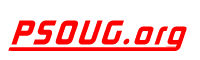Quick Search: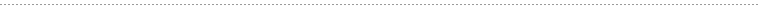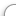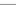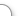CODE Oracle PL/SQL Code Library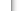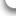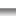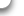JOBS Find Or Post Oracle JobsFORUM Oracle Discussion & ChatThe Oracle PL/SQL MOD Function      [Return To Index] Jump to: Select a Location PSOUG Home Page Oracle Code Library mySQL Code Library PHP Code Library JavaScript Code Library Oracle Terms & Definitions Oracle Error Codes PSOUG Community Blogs Oracle Jobs Board PSOUG Forum Oracle User Group Directory Free Oracle Magazines Online Learning Center PSOUG Presentations Advanced Code Search News and Events Sponsors Page Submit Code Contact UsLooking for the original pages? (formerly called "Morgan's Library") You can find them here.# Term: MOD

Definition:
The Oracle PL/SQL MOD (short for modulus) function returns the remainder when one argument is divided by the second argument. It accepts two number arguments and returns a single numeric value. The modulus is calculated according to the formula: x - y * floor(x/y).

Example Syntax:

`MOD(x,y)`

The remainder will be returned when 'x' is divided by 'y'

The mod function will return x if y is 0. If y = 0, it returns x.

Example Usage:

The SQL below returns the remainder when 10 is divided by 3 (leaving '1').

```SQL> SELECT MOD(10,3) RES FROM DUAL;

RES
----------
1```

The SQL below shows a case where the dividend is less than the divisor.

```SQL> SELECT MOD(3,10) RES FROM DUAL;

RES
----------
3```